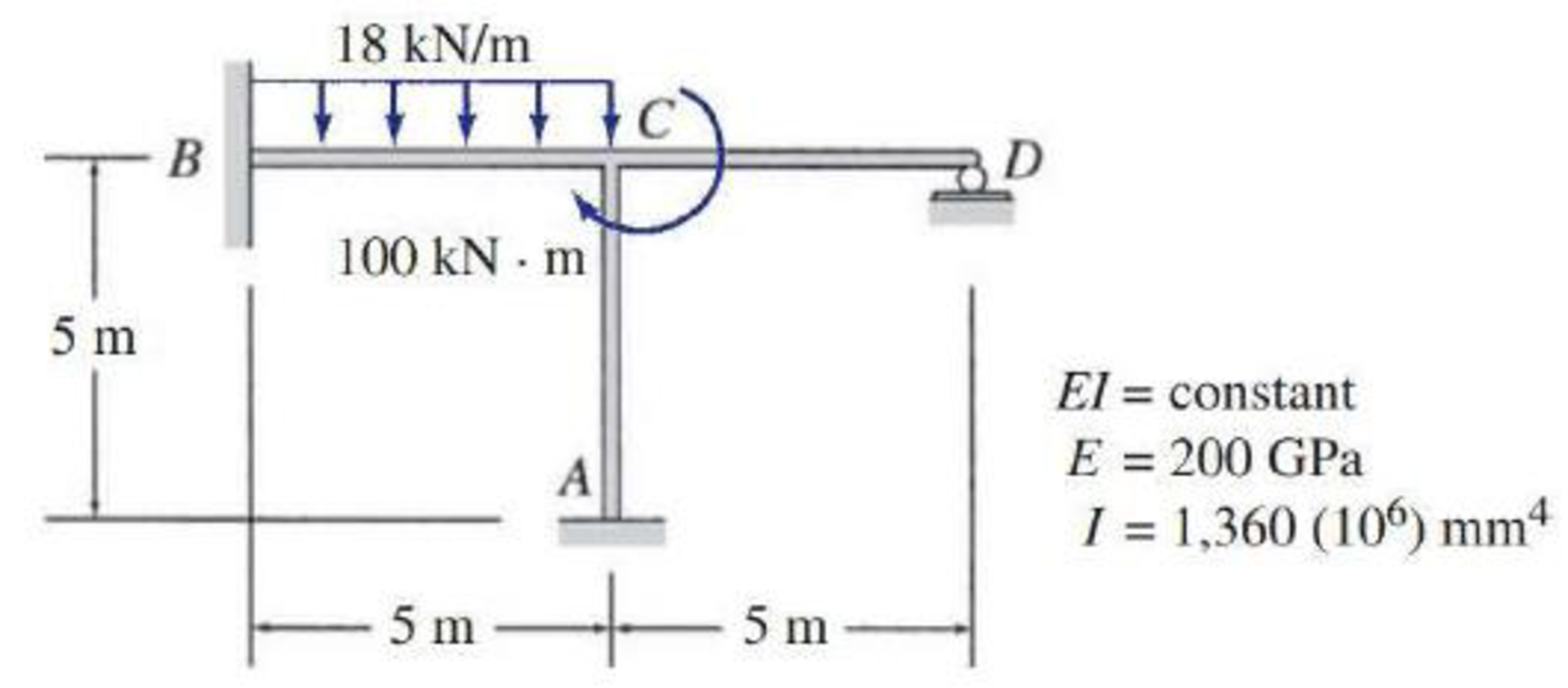# Solve Problem 15.18 for the loading shown in Fig. PlS.18 and a settlement of 6 mm at support A. 15.17 through 15.20 Determine the member end moments and reactions for the frames shown in Figs. P15.17–P15.20 by using the slope-deflection method. FIG. P15.18, P15.22

#### Solutions

Chapter
Section
Chapter 15, Problem 22P
Textbook Problem
93 views

## Solve Problem 15.18 for the loading shown in Fig. PlS.18 and a settlement of 6 mm at support A.15.17 through 15.20 Determine the member end moments and reactions for the frames shown in Figs. P15.17–P15.20 by using the slope-deflection method.FIG. P15.18, P15.22

To determine

Find the member end moments and reaction for the frames.

### Explanation of Solution

Fixed end moment:

Formula to calculate the fixed moment for UDL is WL212.

Calculation:

Consider the flexural rigidity EI of the frame is constant.

Show the free body diagram of the entire frame as in Figure 1.

Refer Figure 1,

Calculate the fixed end moment for BC.

FEMBC=18×(5)212=37.5kNm

Calculate the fixed end moment for CB.

FEMCB=37.5kNm

Calculate the fixed end moment for AC.

FEMAC=0kNm

Calculate the fixed end moment for CA.

FEMCA=0kNm

Calculate the fixed end moment for CD.

FEMCD=0kNm

Calculate the fixed end moment for DC.

FEMDC=0kNm

Chord rotations:

Show the free body diagram of the chord rotation of the frame as in Figure 2.

Calculate the chord rotation of the frame BC.

ψBC=6mm×1m1000mm5m=0.0012

Calculate the chord rotation of the frame CD.

ψCD=6mm×1m1000mm5m=0.0012

Calculate the slope deflection equation for the member AC.

MAC=2EIL(2θA+θC3ψ)+FEMAC

Substitute 200 GPa for E, 1360×106mm4 for I, 0 for ψ, 0 for θA, 5 m for L and 0kNm for FEMAC.

MAC=2×200×13605(2(0)+θC3(0))+0=108800θC        (1)

Calculate the slope deflection equation for the member CA.

MCA=2EIL(2θC+θA3ψ)+FEMCA

Substitute 200 GPa for E, 1360×106mm4 for I, 0 for ψ, 0 for θA, 5 m for L and 0kNm for FEMCA.

MCA=2×200×13605((0)+2θC3(0))0=217600θC        (2)

Calculate the slope deflection equation for the member BC.

MBC=2EIL(2θB+θC3ψBC)+FEMBC

Substitute 200 GPa for E, 1360×106mm4 for I, -0.0012 for ψBC, 0 for θB, 5 m for L and 37.5kNm for FEMBC.

MBC=2×200×13605(0+θC(3×0.0012))+37.5=108800θC+391.7+37.5=108800θC+429.2        (3)

Calculate the slope deflection equation for the member CB.

MCB=2EIL(2θC+θB3ψCB)+FEMCB

Substitute 200 GPa for E, 1360×106mm4 for I, -0.0012 for ψCB, 0 for θB, 5 m for L and -37.5kNm for FEMCB.

MCB=2×200×13605(0+2θC3(0.0012))37.5=217600θC+391.737.5=217600θC+354.2        (4)

Calculate the slope deflection equation for the member CD.

MCD=3EIL(θC+2θDψCD)+FEMCD

Substitute 200 GPa for E, 1360×106mm4 for I, 0.0012 for ψCD, 0 for θD, 5 m for L and 0kNm for FEMCD.

MCD=3×200×13605(0+θC(0.0012))+0=163200θC195

### Still sussing out bartleby?

Check out a sample textbook solution.

See a sample solution

#### The Solution to Your Study Problems

Bartleby provides explanations to thousands of textbook problems written by our experts, many with advanced degrees!

Get Started

Find more solutions based on key concepts
Explain what is meant by an isometric drawing.

Engineering Fundamentals: An Introduction to Engineering (MindTap Course List)

What is a qualitative risk assessment?

Management Of Information Security

What is cloud computing, and why is it a game changer?

Database Systems: Design, Implementation, & Management

How do ultrathin laptops differ from traditional laptops? (111)

Enhanced Discovering Computers 2017 (Shelly Cashman Series) (MindTap Course List)

What is data scrubbing?

Fundamentals of Information Systems

Why is it unsafe to carry butane lighters or matches in your pockets while welding?

Welding: Principles and Applications (MindTap Course List)

If your motherboard supports ECC DDR3 memory, can you substitute non-ECC DDR3 memory?

A+ Guide to Hardware (Standalone Book) (MindTap Course List)